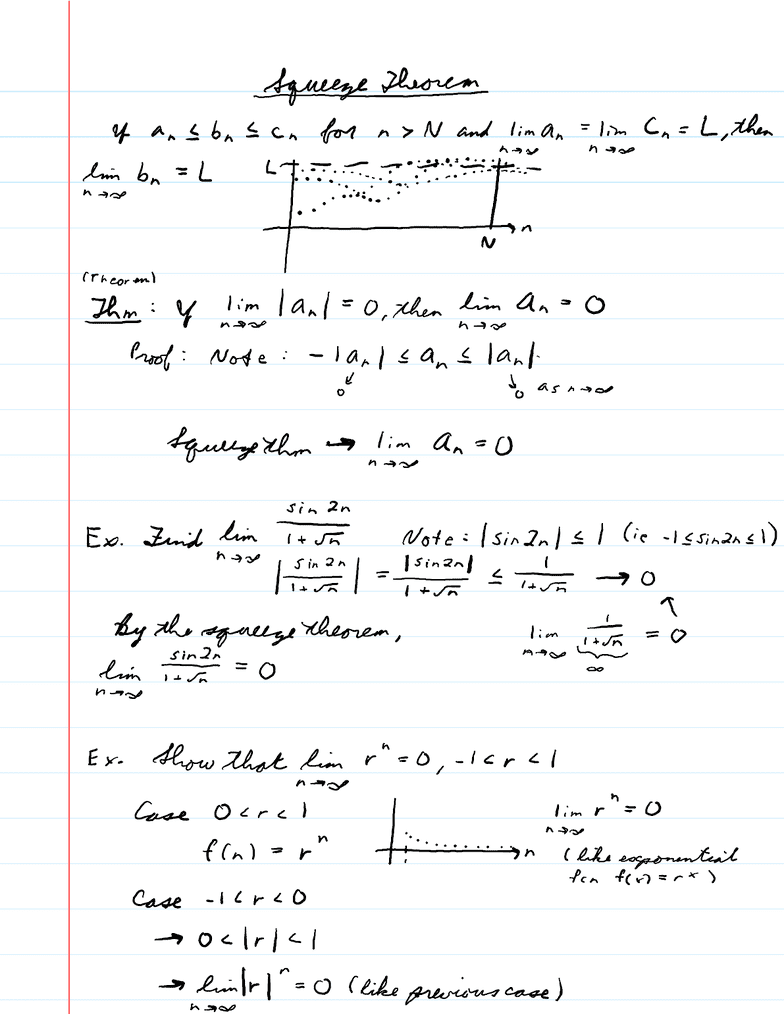Class Notes (1,000,000)
CA (610,000)
U of A (10,000)
MATH (1,000)
MATH100 (300)
Lecture 5

MATH 100 Lecture 5: 5_Squeeze TheoremMonotonic Theorem

Department
Mathematics
Course Code
MATH100
Professor
Lecture
5

This preview shows page 1. to view the full 4 pages of the document.Squeeze Theorem/Monotonic Theorem
September 16, 2015
10:53 AM
Daily Notes Page 1